# 5 NEUTRINOS AS OSCIS AND MORE - Die eine Quantentheorie

Die eine Quantentheorie
Menue in German and English - select!
Es geht um die einfachste Stringtheorie nach Art der ART,
die den Aufbau der Elementarteilchen erklärt.
Phänomene und Paradoxa lassen sich math. begründen.
Benutzt werden Falsifikation und das Noether-Theorem
mit der letzten Invarianz der Quanten.

It's about the simplest string theory in the way of the GR,
which
explains the structure of the elementary particles.
Phenomena and paradoxes can be justified mathematically.
Used are falsification and the Noether theorem with
the last invariance of the quanta.
The one quantum theory
Direkt zum Seiteninhalt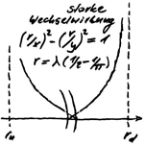Diese Seite ist
frei von Esoterik
und Quantenlogik!
Die Theoie
der Oszis

## 5 NEUTRINOS AS OSCIS AND MORE

ENGLISH ▶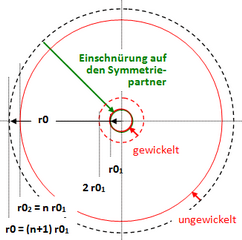The elementary particles must result as a solution to the variation problem. This refers to a pair of oscis that satisfies the general symmetry, where the symmetry energy is known as the conserved quantity. The solutions are where the energy of the elementary particle has a local minimum summed over all binding energies. This problem can be solved computationally, because besides the general symmetries other symmetries and exchange rules apply. If the calculation of the total energy of the found pair does not add up, it has to be clarified which energy form(s) are in the rest.

Only the osci that has more than one turn in the plane of the E-field (strong interaction with itself) can be considered as the neutrino-osci. The winding type is thus that of a short-circuit coil, where start and end are connected without twisting the field. The dipole wave is folded accordingly often (synchronicity). Charge and spin are thus 0, which means that the electromagnetic binding energy is omitted. As always, the conserved quantity is the symmetry energy. Index 1 in the pair of oszis stands for the boson and index 2 for the neutrino. The sketch on the right shows how the constriction of the neutrino circular wave takes place - more about the calculation in the PDF.

(1) The variation problem can only be solved if the corresponding boson is destroyed.
(2) However, with (1) the energy of the neutrinos becomes negative.
(3) Solutions (local minima) exist for n = 2 to 21929645 windings - see table.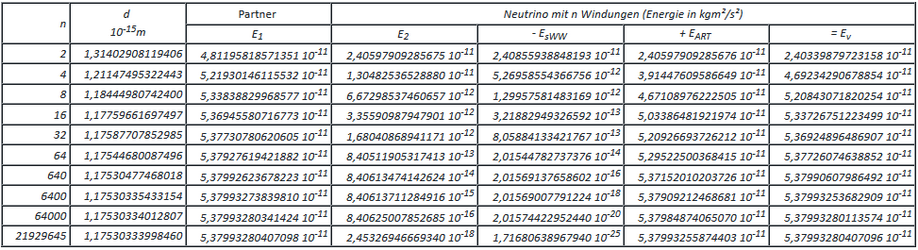If the energy balance is to be correct, is Ekin = E1 + E2 - Eν =  EsWW + E2 = EsWW + E1 - EART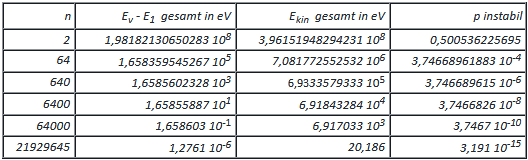With the instability from the above table, the experiments on neutrino oscillation become plausible. The results of the neutrino-oscillations lead to a picture of the universe that finally corresponds to the observations. In addition, there are a number of predictions!

First the hint that there is no zero point fluctuation in the TO. Thus the negative energy of the neutrinos (2) can only be compensated by kinetic energy due to the lack of an alternative, so that it is only massless at c! The reason is the non-existent zero point fluctuation - see also INTERPRETATION OF THE MASS. Since, on the other hand, it has a mass defect, both experimental physics and theoretical physics are right. (3) has its reason in the necessary connection of the beginning and end of the circular wave, which at some point can no longer be realized with the allowed curve. So at plane of E-field must result minimum radius. This can be calculated, because the upward oscillation is convergent - see table (more in PDF).

The geometry of the neutrinos: The rotation of the circular wave in relation to the flight direction is the helicity of the neutrinos. The propagation line of the wave within the neutrinos results in a coil (short circuit coil). Chirality occurs when a coil wound to the left or right is combined with the same or opposite direction of rotation of the shaft. Since the direction of rotation of the shaft is inherited from the primitive oscillator, it also determines the winding direction of a developing coil. According to the rule of thumb, it is left-handed, which also determines the direction of escape.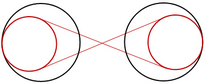The pre-school experiment: The minimum radius of 0,5876516699923 10-15 m can also be reached via the osci-eight of the down-osci. If the eight is stretched over the minimum radius, the Oszi circles slide apart to the range of the strong interaction. This leads to the conclusion that the down-oszi is the oszi with the highest energy. His Boson is the up-osci. All other bosons are more energetic and the perturbation continues to increase.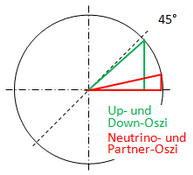Within unit circle right side, two angles fall into eye. The angle difference of the up- and down-oscis to 45° is 0,093534930234064000°. With all symmetry this is the existentially necessary asymmetry! The other angle results with the lowest energy neutrino. The angle difference to 0 there is about 2,58°, which corresponds to a mass gap of approx. 2,246 10-16 eV/c². From the angular relation in the Thales circle to its boson, the stretching factor of the space can be determined. With it, a background temperature of TH = 0,000182223 K results from the Beckenstein-Hawking entropy. The calculation assumes that the empty universe already has an event horizon. The absolute quantum vacuum starts with the temperature TH , so that the straight line with V/T = const. must be extended downwards beyond V = 0, to V = -6,67117918 10-7.

The universe is prestressed, which explains the dark energy.
With the principle of constriction, matter has to flee ever faster to the event horizon.

If n = 21929645, it's not really over. The geometry tilts there. The circular wave becomes a donate and the dipole wave arranges its folding in a circle. So it can go on. There is no longer a back, i.e. a downward oscillation, since the instability decreases abruptly with the transition, by a factor of 1/π, with which it goes towards 0. The threshold to Dark Matter is thus found. The D-Neutrinos (D of Donat) are absolutely rotationally symmetric. They are the presumed sterile neutrinos, since a phase shift around π at one wavelength (= 2π) is synonymous with a reversal of the direction of rotation, which can also be regarded as a time reversal. On the other hand, due to the rotational symmetry of the torus, it does not matter where the zero crossings of the shaft are. With the transition to the D-neutrino not only the minimum radius of curvature in the plane of the E-field is known, but also the packing density (a, b) in both field directions.

b = ½ · 7,67779726787773 10-30 m in the E-Feld,
a = ½ · 5,35942711331898 10-23 m in the B-Feld,
so also minimum radius of curvature within B-field is known (theoretic 0).
The "principle of constriction" leads to the separation of the energy density on the edge. If density is represented as a function, the question of its continuity arises.The function can be continuously connected on the edge if there is an open environment at each edge point that can be continuously embedded. For this environment the elliptical cross section above is suitable. The synchronous constriction of circle and dipole wave leads, according to the "prediction of TO", specifically to its gravitational decoupling. Thus there must be an envelope which connects the points of the circular wave and the end points of the dipole wave on the way of minimum space stress.

A further conclusion can be drawn from the convergence of the upward oscillation of the neutrinos. At the transition to the D-neutrino the following energy density results for the circular wave:

w = E/r = 5,379933... 10-11 (kgm²/s²)/1,288699... 10-8 m

With the energy of the circular wave also the energy of the dipole wave is known. It has to be multiplied by 2/π², because on the one hand the reference radius (0-radius) is greater by π/2. Since it is in the denominator, the reciprocal is to be taken. On the other hand, the factor 1/π results from the "energy equation of the osci". The decoupling of the space-time plane or the space-time line as a whole is now decisive. Since the energy densities remain constant with decoupling, normalization is permitted - times 1/[m]. This results in the following values in the empty universe:

-5,37993304939792 10-11 kgm/s² for the space-time plane and
-1,09020236896306 10-11 kgm/s² for the space-time line.

The prestress values are negative. In empty space without any space-time deformation, the total stress results simply from its addition. This can be justified by the covariance of the Cartesian coordinates, which applies at least locally in the ART. Looking at the law of gravity F = G m1 m2/(-r²) in relation to its units, the masses are sample masses of 1kg, and r is one meter. This gives the gravitational constant of the empty universe:

G00 = -6,47013541836098 10-11 m³/kgs²

If G is replaced by the above constant in Einstein's field equations, the GR describes the space-time continuum as empty space. It is he who gives mass to the osci. It will be shown that this is also the case with the photon - see SPOOKY PHOTON.

The difference of the universal gravitational constant from G of about 3.1521% does not contradict the measurements of astrophysics. They predict about 4.2% baryonic matter, whereby still the "cold dark matter" has to be considered. Because if I have calculated correctly, only about every 4.62nd neutrino survives the upward oscillation up to the D-neutrino. The D-Neutrinos form very firm stuffing places, so it should not be surprising that the universe gets cellulite, also known as filaments. Relativistic dark matter (hot dark matter) thus becomes non-relativistic dark matter (cold dark matter). This lowers the internal tension of the space in its environment, with which the self-organization might begin. The result is a space lattice of cold dark matter.
last modification 10.03.2019
go to side  1  2  3  4  5  6  7  8  9
Zurück zum Seiteninhalt